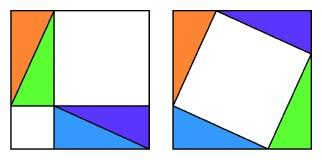## Diagonals of Cubes in Different Dimensions

We get a different sort of pattern when we analyze the lengths of diagonals of cubes in different dimensions. A diagonal of a cube is a segment joining two points that are not the endpoints of an edge. Whereas both diagonals of a square have the same length, a cube has diagonals of two different lengths, the shorter ones lying on square faces and the longer ones passing through the center. In each dimension, we may compare the length of the side of a cube to the length of its longest diagonal, thus obtaining another significant pattern.The longer and shorter diagonals of a cube are the hypotenuse and edge of a right triangle.

Each time a cube is formed in a new dimension, it means adding edges in a direction perpendicular to all of the directions thus far. The final edge is perpendicular in particular to the longest diagonal of the previous cube, and these two segments form the two sides of a right triangle having the longest diagonal of the new cube as its hypotenuse. This suggests that we can find the length of the longest diagonal by using one of the most famous of all theorems in plane geometry, the Pythagorean theorem.

This theorem states that in a right triangle having sides of length p and q, the length of the hypotenuse is (p2 + q2)1/2. The decomposition we found in interpreting the binomial theorem leads to a proof of this theorem by showing that the area of a square on the hypotenuse of a right triangle is the sum of the areas of the squares on these sides. To see this, we express the area of a square of side length p + q in two ways: once as the sum of the areas of two squares of side lengths p and q and of four right triangles of side length p and q, and again as the sum of the areas of the same four right triangles together with the square bounded by the hypotenuses of those triangles of side length (p2 + q2)1/2.

Applying the Pythagorean theorem to the right triangles formed by cube diagonals sets up an easily discerned pattern. For any dimension, we consider a cube having side length 1. For a square, when n = 2, the diagonal has length Ö2. For a cube, when n = 3, the diagonal will be the hypotenuse of a right triangle with base square root of 2 and height 1, and by the Pythagorean theorem, the length of the diagonal will be

 Ö ________ Ö _____ Ö __ (Ö2)2 + 12 = 2 + 1 = 3 .

Moving to the four-dimensional hypercube, we obtain a new right triangle with base of length Ö3 and height 1, therefore with hypotenuse
 Ö _________ Ö _____ Ö __ (Ö3)2 + 12 = 3 + 1 = 4 = 2.

Thus the hypercube has a diagonal exactly twice the length of a side. It is easy to see that, in general, the length of the longest diagonal of an n-dimensional cube will be Ön, and this is quickly proved by mathematical induction: if we already know that the length of the diagonal of an (n-1)-cube is square root of n-1, then the diagonal of the n-cube is the hypotenuse of a right triangle with one side of length Ö(n-1) and the other of length 1. By the Pythagorean theorem, we have
 Ö ______________ Ö ________ Ö __ (Ö(n-1))2 + 12 = n-1 + 1 = n

as the length of the diagonal of the n-cube of side length 1.A demonstration of the Pythagorean theorem. Two squares built on the edges of a right triangle and four copies of the triangle exactly fill a large sqwuare that is also filled by four copies of the triangle and a square built on the hypotenuse. Take out the triangles, and we have the squares built on two sides of the triangle equal in area to the area of the square built on the hypotenuse.

When we compare a unit cube to a cube of side length m, we observe that the lengths of the diagonals of the new cube are an times as large as the corresponding diagonals of the unit cube. Therefore the length of the longest diagonal of an n-cube of side length m is mÖn, and the dimension appears under the square root sign. Note that the longest diagonal of a four-cube will be exactly twice as long as one of its edges.